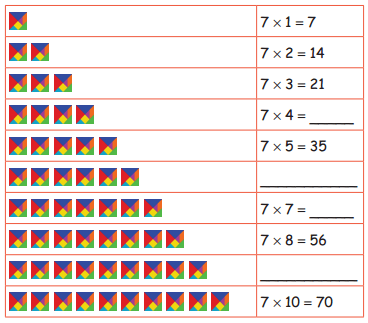Students can download 4th Maths Term 2 Chapter 2 Numbers Ex 2.2 Questions and Answers, Notes, Samacheer Kalvi 4th Maths Guide Pdf helps you to revise the complete Tamilnadu State Board New Syllabus, helps students complete homework assignments and to score high marks in board exams.

## Tamilnadu Samacheer Kalvi 4th Maths Solutions Term 2 Chapter 2 Numbers Ex 2.2

Question 1.
4 × 6 = _____
4 × 6 =  24Question 2.
7 × 2 = ______
7 × 2 =  14

Question 3.
8 × 2 = ______
8 × 2 =   16Question 4.
In a shirt there are 6 buttons, how many buttons will there be in 8 shirts?
No. of button in a shirt = 6
No. of button in 8 shirts = 6 × 8
= 48 button

Question 5.
In a carton, there are 9 rows with 6 eggs in each row. How many eggs are there in all?